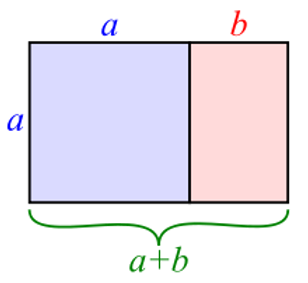## Golden Ratio and the inheritance injustice to womenQURAN 4.11

..... The male shall have

the equal of the portion of two females.....

Based on the above ayat, with the current distribution of inheritance

the man gets 66.6%

and the woman 33.3%.

Calculated at a 2:3 male Ratio

Actually with close inspection, the ayat states the EQUAL portion of two females.

The Quran is mentioning "the Golden ratio", which is calculated differently to the current 2:3 ratio.

The Golden Ratio is calculated at a 2:1 ratio

Two quantities are in the golden ratio if their ratio is the same as the ratio of their sum to the larger of the two quantities - 1.618

This would meanMen should get 61.80% (instead of 66.6%)

Women should get 38.20% (instead of 33.3%)

an injustice to women, by 4.85%